# T3 Coordinates (continued)

On one accompanying wiki page we have introduced T3 Coordinates and on another we have described how Jay Call's Characteristic Vector applies to T3 Coordinates. Here we investigate the properties of our T3 Coordinate system in the special case when q2 = 2; Jay Call's independent analysis is recorded on a separate page.

### Coordinate Relations

When q2 = 2, the two key coordinates are:

 λ1 =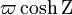$\varpi \cosh\Zeta$ λ2 =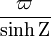$\frac{\varpi}{\sinh\Zeta}$ Note also: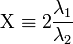$\Chi \equiv 2\frac{\lambda_1}{\lambda_2}$ = 2sinhΖcoshΖ = sinh(2Ζ),

where, in this case,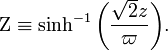$\Zeta \equiv \sinh^{-1} \biggl( \frac{\sqrt{2}z}{\varpi} \biggr) .$

For this special case, we can invert these coordinate relations to obtain analytic expressions for both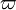$\varpi$ and z in terms of λ1 and λ2. Specifically, the relation,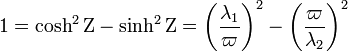$1 = \cosh^2\Zeta - \sinh^2\Zeta = \biggl(\frac{\lambda_1}{\varpi}\biggr)^2 - \biggl(\frac{\varpi}{\lambda_2}\biggr)^2$

implies that the function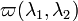$\varpi(\lambda_1,\lambda_2)$ can be obtained from the physically relevant root of the following equation: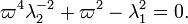$\varpi^4 \lambda_2^{-2} + \varpi^2 - \lambda_1^2 = 0.$

The relevant root gives,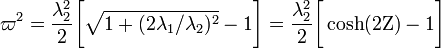$\varpi^2 = \frac{\lambda_2^{2}}{2} \biggl[ \sqrt{1 + (2\lambda_1/\lambda_2)^2} - 1 \biggr] = \frac{\lambda_2^{2}}{2} \biggl[ \cosh(2\Zeta) - 1 \biggr]$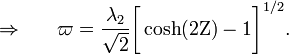$\Rightarrow ~~~~~\varpi = \frac{\lambda_2}{\sqrt{2}}\biggl[ \cosh(2\Zeta) - 1 \biggr]^{1/2} .$

The desired function z12) is therefore,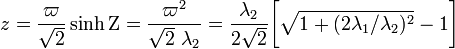$z = \frac{\varpi}{\sqrt{2}} \sinh\Zeta = \frac{\varpi^2}{\sqrt{2}~\lambda_2} = \frac{\lambda_2 }{2\sqrt{2}} \biggl[ \sqrt{1 + (2\lambda_1/\lambda_2)^2} - 1 \biggr]$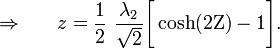$\Rightarrow ~~~~~ z = \frac{1}{2}~\frac{\lambda_2 }{\sqrt{2}} \biggl[ \cosh(2\Zeta) - 1 \biggr] .$

In an effort to simplify the appearance of these and future expressions, we will henceforth adopt the notation,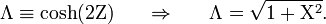$\Lambda \equiv \cosh(2\Zeta) ~~~~~ \Rightarrow ~~~~~ \Lambda = \sqrt{1 + \Chi^2} .$

In terms of Λ, then, we have,$\varpi$ =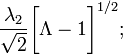$\frac{\lambda_2 }{\sqrt{2}} \biggl[ \Lambda - 1 \biggr]^{1/2} ;$ z =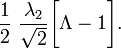$\frac{1}{2}~\frac{\lambda_2 }{\sqrt{2}} \biggl[ \Lambda - 1 \biggr] .$

### Scale Factor Expressions

We are now in a position to express the two key scale factors purely in terms of the two key T3 coordinates. First, we note that,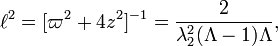$\ell^{2} = [\varpi^2 + 4z^2]^{-1} = \frac{2}{\lambda_2^2(\Lambda - 1)\Lambda} ,$

and,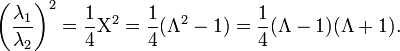$\biggl(\frac{\lambda_1}{\lambda_2}\biggr)^2 = \frac{1}{4}\Chi^2 = \frac{1}{4}(\Lambda^2 -1) = \frac{1}{4}(\Lambda -1)(\Lambda +1) .$

Hence,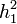$h_1^2$ =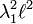$\lambda_1^2 \ell^2$ =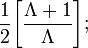$\frac{1}{2}\biggl[ \frac{\Lambda + 1}{\Lambda} \biggr] ;$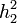$h_2^2$ =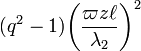$(q^2-1)\biggl( \frac{\varpi z \ell}{\lambda_2} \biggr)^2$ =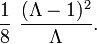$\frac{1}{8}~ \frac{(\Lambda - 1)^2}{\Lambda} .$

We note also that,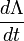$\frac{d\Lambda}{dt}$ =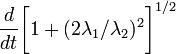$\frac{d}{dt}\biggl[ 1+(2\lambda_1/\lambda_2)^2 \biggr]^{1/2}$ =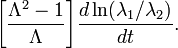$\biggl[ \frac{\Lambda^2-1}{\Lambda} \biggr] \frac{d\ln(\lambda_1/\lambda_2)}{dt} .$

Hence, starting from the general expression for dlnh2 / dt derived elsewhere, we deduce that,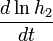$\frac{d\ln h_2}{dt}$ =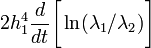$2 h_1^4\frac{d}{dt}\biggl[ \ln(\lambda_1/\lambda_2) \biggr]$ =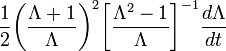$\frac{1}{2} \biggl( \frac{\Lambda +1}{\Lambda} \biggr)^2 \biggl[ \frac{\Lambda^2-1}{\Lambda} \biggr]^{-1} \frac{d\Lambda}{dt}$ =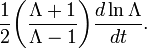$\frac{1}{2} \biggl( \frac{\Lambda +1}{\Lambda-1} \biggr) \frac{d\ln\Lambda}{dt} .$

(This identical expression also can be derived straightforwardly from the specific expression for h2 given above.) From the expression given above for h1, we also deduce that,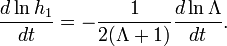$\frac{d\ln h_1}{dt} = - \frac{1}{2(\Lambda + 1)} \frac{d\ln\Lambda}{dt} .$

As a check, we note that the relationship between dlnh1 / dt and dlnh2 / dt in this specific case (i.e., q2 = 2) matches the general relationship between these two logarithmic time-derivatives that has been derived elsewhere, namely,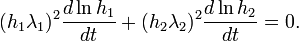$(h_1 \lambda_1)^2 \frac{d\ln h_1}{dt} + (h_2 \lambda_2)^2 \frac{d\ln h_2}{dt} = 0 .$

### Equation of Motion

According to Equation EOM.01, as derived elsewhere in the context of T3 coordinates, a general expression for the second component of the equation of motion is,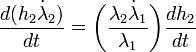$\frac{d(h_2 \dot{\lambda}_2)}{dt} = \biggl(\frac{\lambda_2 \dot{\lambda}_1}{\lambda_1}\biggr) \frac{dh_2}{dt}$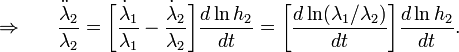$\Rightarrow ~~~~~ \frac{\ddot{\lambda}_2}{\lambda_2} = \biggl[\frac{\dot{\lambda}_1}{\lambda_1} - \frac{\dot{\lambda}_2}{\lambda_2} \biggr]\frac{d\ln h_2 }{dt} = \biggl[\frac{d\ln(\lambda_1/\lambda_2)}{dt} \biggr]\frac{d\ln h_2 }{dt} .$

Based on the derivations provided above, both factors that make up the term on the RHS of this expression can be written entirely in terms of the variable, Λ. This allows us to rewrite Equation EOM.01 as,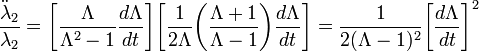$\frac{\ddot{\lambda}_2}{\lambda_2} = \biggl[\frac{\Lambda}{\Lambda^2-1} \frac{d\Lambda}{dt}\biggr]\biggl[ \frac{1}{2\Lambda} \biggl( \frac{\Lambda + 1}{\Lambda - 1} \biggr) \frac{d\Lambda}{dt} \biggr] = \frac{1}{2(\Lambda - 1)^2} \biggl[\frac{d\Lambda}{dt} \biggr]^2$

T3Q.01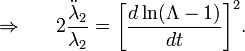$\Rightarrow ~~~~~ 2\frac{\ddot{\lambda}_2}{\lambda_2} = \biggl[\frac{d\ln(\Lambda-1)}{dt} \biggr]^2.$

### Solution Strategy

I'm not sure whether the following strategy is fully legitimate, but let's explore it anyway. Because the LHS of Equation T3Q.01 displays an explicit dependence only on the coordinate λ2 while the RHS displays an explicit dependence only on Λ — that is, only on the ratio of the two coordinates λ1 / λ2 — perhaps we can use a separation of variables technique to derive a solution. Specifically, suppose the LHS and the RHS separately are set equal to the same value, call it n.

Then, for the LHS: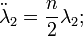$\ddot{\lambda}_2 = \frac{n}{2}\lambda_2 ;$

And, for the RHS: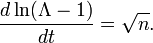$\frac{d\ln(\Lambda-1)}{dt} = \sqrt{n} .$

Now I suppose that, in general, n should be allowed to vary with time, but for exploratory purposes, let's assume that n is a constant. The solution to the LHS's 2nd-order ODE is,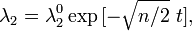$\lambda_2 = \lambda^0_2 \exp{[-\sqrt{n/2}~t]} ,$

where,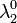$\lambda^0_2$ is the coordinate position λ2 at time t = 0. The solution to the RHS's 1st-order ODE is,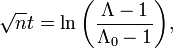$\sqrt{n} t = \ln\biggl( \frac{\Lambda-1}{\Lambda_0 -1} \biggr) ,$

where, Λ0 is given by the coordinate ratio at time t = 0, specifically,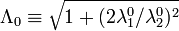$\Lambda_0 \equiv \sqrt{1 + (2\lambda_1^0/\lambda_2^0)^2}$.

Now, if we replace "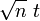$\sqrt{n}~t$" in the first of these expressions by the second expression, we find,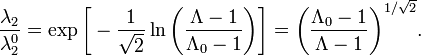$\frac{\lambda_2}{\lambda^0_2} = \exp{\biggl[ -\frac{1}{\sqrt{2}} \ln\biggl( \frac{\Lambda-1}{\Lambda_0 -1} \biggr) \biggr]} = \biggl( \frac{\Lambda_0 -1}{\Lambda-1} \biggr)^{1/\sqrt{2}} .$

This would be a fantastically simple result, if it proved to be a proper solution to the governing equation of motion. Unfortunately, if this relatively elementary equation is differentiated twice in an effort to reproduce Equation T3Q.01, we find that an additional undesirable term appears that involves the second derivative of a function containing the variable Λ. It can be shown that this undesirable term goes to zero if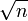$\sqrt{n}$ is assumed to be independent of time (as we did indeed assume, above). Unfortunately, in reality, this does not seem to be a desirable assumption for the physical problem in which we have interest, so we must conclude that the derived elementary equation is not the desired solution of the equation of motion.

But can we learn something valuable from this failed separation of variables approach???

### Another Thought

It can easily be shown that, in general,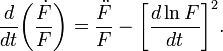$\frac{d}{dt}\biggl( \frac{\dot{F}}{F} \biggr) = \frac{\ddot{F}}{F} - \biggl[\frac{d\ln F}{dt} \biggr]^2 .$

Hence, Equation T3Q.01 can be rewritten as,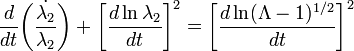$\frac{d}{dt}\biggl( \frac{\dot{\lambda_2}}{\lambda_2} \biggr) + \biggl[\frac{d\ln \lambda_2}{dt} \biggr]^2 = \biggl[\frac{d\ln (\Lambda-1)^{1/2}}{dt} \biggr]^2$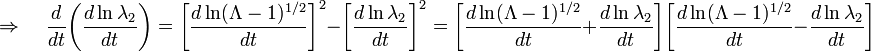$\Rightarrow ~~~~~ \frac{d}{dt}\biggl( \frac{d\ln\lambda_2}{dt} \biggr) = \biggl[\frac{d\ln (\Lambda-1)^{1/2}}{dt} \biggr]^2 - \biggl[\frac{d\ln \lambda_2}{dt} \biggr]^2 = \biggl[\frac{d\ln (\Lambda-1)^{1/2}}{dt} + \frac{d\ln \lambda_2}{dt} \biggr] \biggl[\frac{d\ln (\Lambda-1)^{1/2}}{dt} - \frac{d\ln \lambda_2}{dt} \biggr]$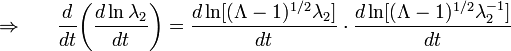$\Rightarrow ~~~~~ \frac{d}{dt}\biggl( \frac{d\ln\lambda_2}{dt} \biggr) = \frac{d\ln [(\Lambda-1)^{1/2}\lambda_2]}{dt} \cdot \frac{d\ln [(\Lambda-1)^{1/2} \lambda_2^{-1}]}{dt}$

Can we gain any insight from this form of the 2nd component of the equation of motion? For example, can the convert the RHS into the total time-derivative of some function?

 © 2014 - 2020 by Joel E. Tohline |   H_Book Home   |   YouTube   | Appendices: | Equations | Variables | References | Ramblings | Images | myphys.lsu | ADS |